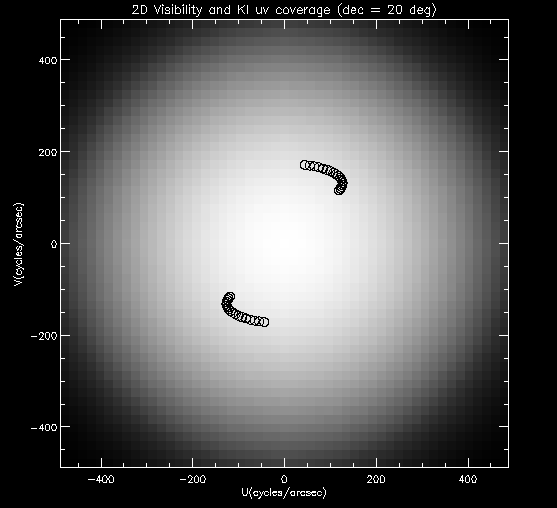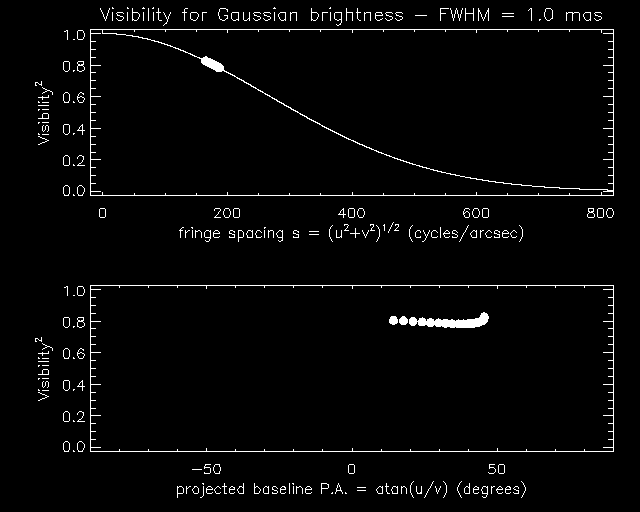# Keck Interferometer Tutorial: What is a visibility?

The visibility is the fundamental observable of the KI in the operational mode refered to as "visibility amplitude" (a.k.a. "visibility squared" or V2 mode). As explained in the references and tutorials given in our basic bibliography list, an interferometer measures discrete components of the spatial frequency spectrum of the observed object's brightness distribution on the sky. This spatial spectrum is often called the "visibility function", a complex function in the general case. The spatial frequencies sampled are a function of the locations of the interferometer's apertures, the location of the source in the sky, and the wavelength of observation.

When observing from the ground, the phase of the spatial spectrum is; corrupted by atmospheric turbulence, and is not recoverable using closure phase techniques for a two-telescope interferometer, such as the KI. Therefore, the useful observable is the modulus of the spatial spectrum. Also, with only two apertures, the KI can only obtain a relatively sparse sampling of spatial frequencies for a given object. Therefore, imaging by Fourier-inversion techniques (common in radio-interferometry) is not possible.

Nevertheless, an optical interferometer such as the KI can offer important advantages over a conventional telescope. This is because the visibility amplitudes measured correspond to the very high spatial resolution given by the large relatively separation between the apertures (or baseline length); analogous to the resolving power of a conventional telescope being given by the aperture diameter. As an approximate rule, the maximum spatial frequency sampled by an interferometer may be estimated as s(max)=B/wavelength; where B is the length of the baseline vector projected on the sky. At 2.2 microns, and for B=85m, we have s(max)=187 cycles/arcsec. Similarly, a convenient "angular resolution" definition is given by wavelength/B; or 5 milliarcsec for the example chosen above.

Experimentally, an interferometer measures components of the visibility function by forming interference fringes between the apertures. The contrast and phase of these fringes give the amplitude and phase of the visibility function, respectively. In practice, the square of the visibility amplitude is often measured, hence the nomenclature for the mode. In reality, the atmosphere and instrument degrade the ideal measurement, in a way that can be easily calibrated using measurements of targets of known visibility, such as stars known to appear as point sources or stars of well known angular diameter. Following standard practice in optical interferometry (and contrary to the case of radio-interferometry), in the KI data processing the visibility is measured relative to the mean flux detected. With such normalization, a calibrated visibility of 1.0 is obtained for an object that is unresolved by the KI (a point source), and a visibility of 0.0 would be measured for an object that is completely resolved. For partially resolved sources, a visibility between 0.0 and 1.0 is measured.

Once raw KI visibilities have been measured and calibrated, the astrophysical information about theobject being studied can be extracted using a priori knowledge and model fitting techniques.

Let's consider some simple examples. In Figure 1 we represent an object on the sky that has a Gaussian brightness distribution. Note its very small angular extent, with a full-width at half-maximum of 1 mas.Figure 1. A simple object represented by a Gaussian brightness.

The spatial spectrum of the Gaussian object, or visibility function, is given by the 2D Fourier transform of the sky brightness. The visibility amplitudes (squared) are shown in Figure 2. Overplotted, we show the track of spatial frequencies that would be sampled by the KI if this object were located at a declination of 20 degrees, and observed for an hour angle range of -3 hr -> +3 hr. The time sampling corresponds to a realistic KI data acquisition rate of 3 calibrated scans/hour. If you would like to learn how to calculate the (u,v) spatial frequencies that would be sampled by a given interferometer and given the source coordinates, please consult e.g. the Thompson, Moran & Swenson reference in our bibliography.

Note also that our observations planning tool getCal makes this computation for you.Figure 2. The 2D visibility (squared) map of the Gaussian brightness. The superposed arcs show a realistic KI sampling of spatial frequencies.

Finally, Figure 3 shows the visibility amplitude curve for this object, along with the samples that KI would measure on this object. Since the object is circularly symmetric on the sky, its Fourier transform is also circularly symmetric and in the top panel we represent the visibilities as a function of the modulus of the spatial frequency (u,v) pairs. To further illustrate the geometry, in the bottom panel the visibilities are plotted as a function of the projected baseline position angle. As the Earth rotates the projected baseline may change not only in orientation but also in length, hence even though our source is circularly symmetric, there are small visibility variations seen in the bottom plot.Figure 3. Gaussian source visibility curves and simulated KI samples.
Another simple example is shown in Figure 4. This time we consider a binary stellar system, where the separation between the stars is only 5 mas. This object is not circularly symmetric, and therefore the visibilities measured will depend strongly on the relative orientation of the baseline vector and, in this case, the binary separation vector. Equivalent to baseline position angle, we now show the visibilities as a function of time, or source hour angle, and it can been seen how as the Earth rotates the visibilities trace the sinusoidal response characteristic of a binary system. Note that this variation would take place even if the projected baseline length remained constant over this time interval. As in the previous example, the samples that KI would measure are overplotted, and have been calculated assuming the same source declination and (realistic) sampling intervals.Figure 4. Visibility curve for a binary star and simulated KI samples.

Rafael Millan-Gabet, April 09 2005